# RD Sharma Solutions for Class 10 Maths Chapter 3 Pair of Linear Equations In Two Variables Exercise 3.11

Miscellaneous applications of linear equations are the key focus of this exercise. The RD Sharma Solutions Class 10 developed by experts at BYJU’S is a prime resource for students to clarify their doubts and help them analyse their weaker areas. Further, students can also utilise the RD Sharma Solutions for Class 10 Maths Chapter 3 Pair of Linear Equations In Two Variables Exercise 3.11 PDF given below.

## RD Sharma Solutions for Class 10 Maths Chapter 3 Pair of Linear Equations In Two Variables Exercise 3.11 Download PDF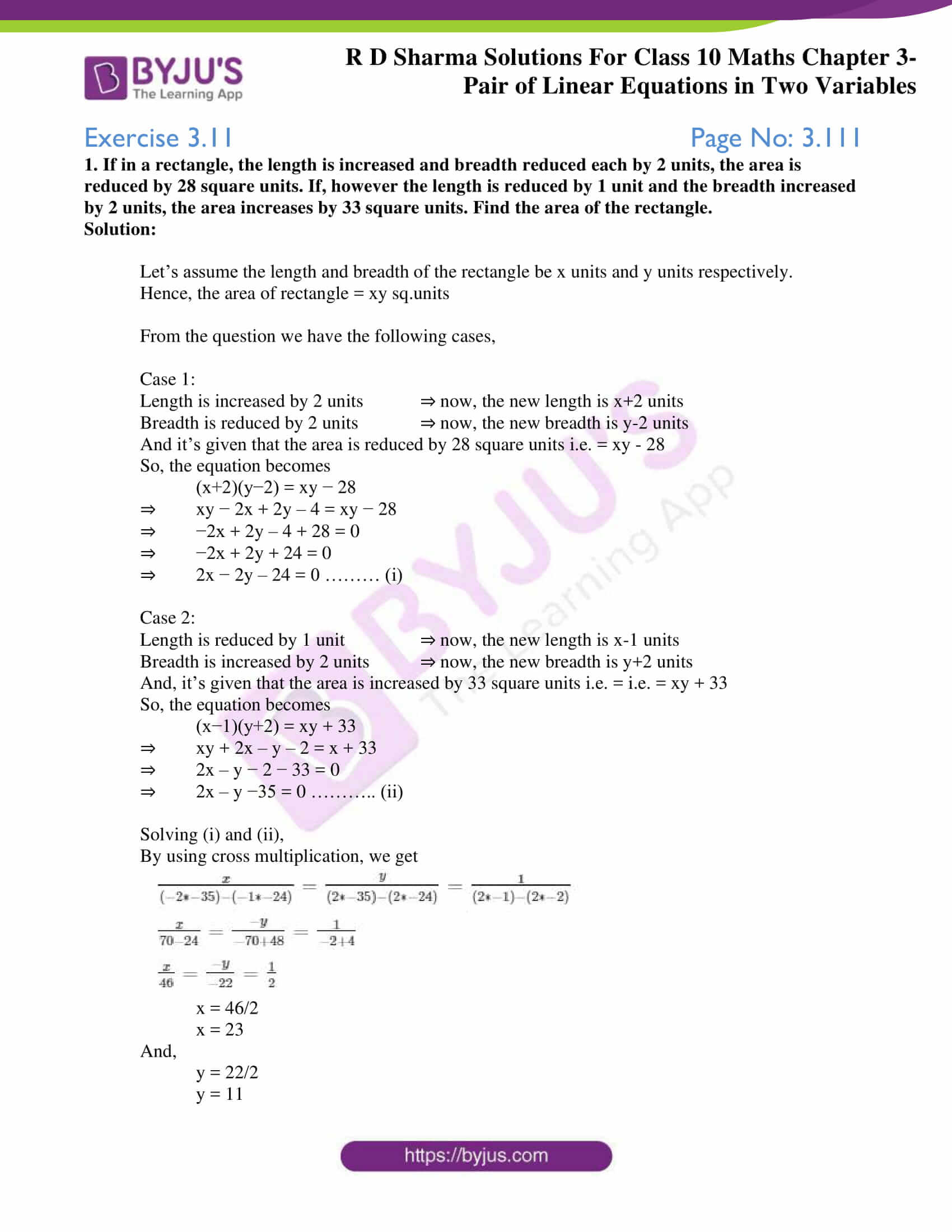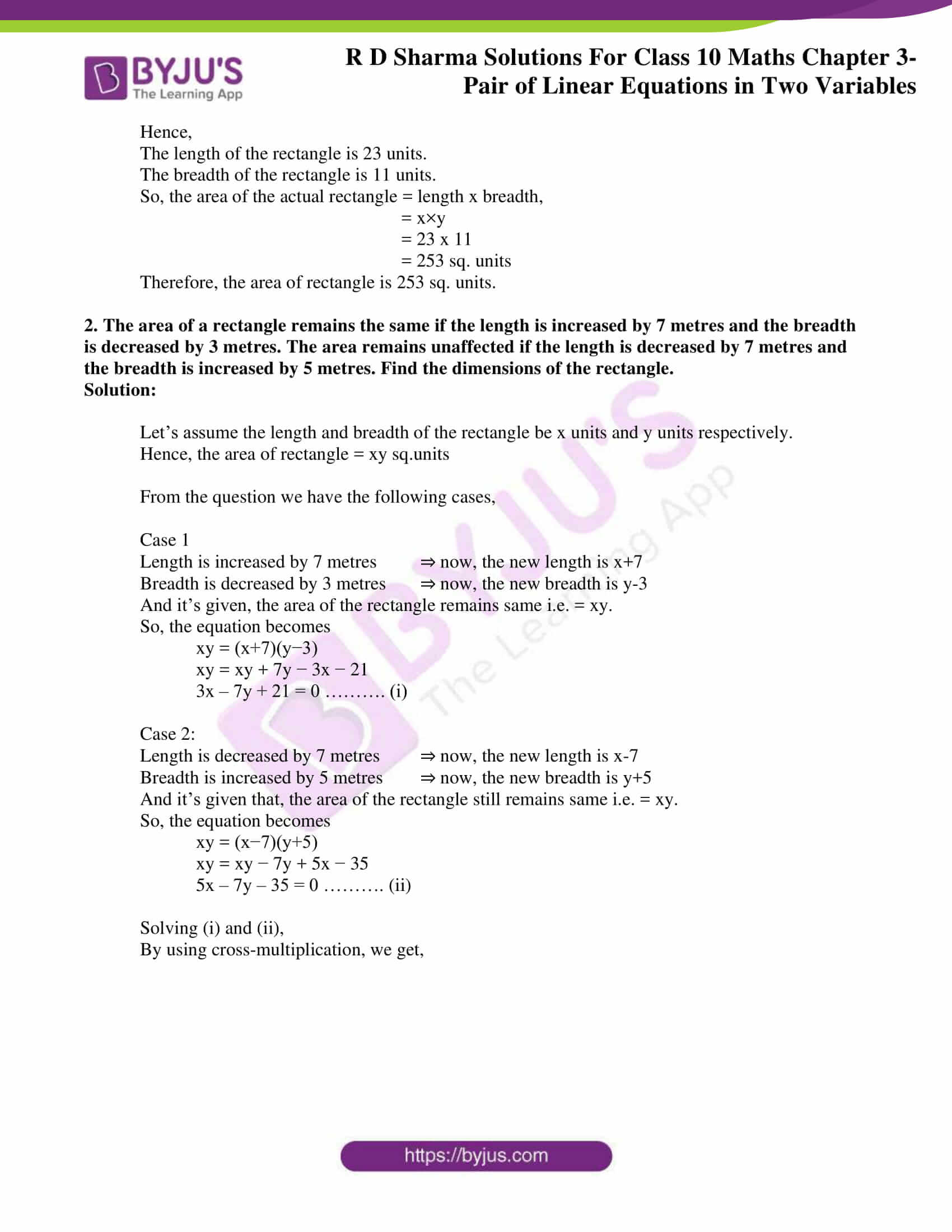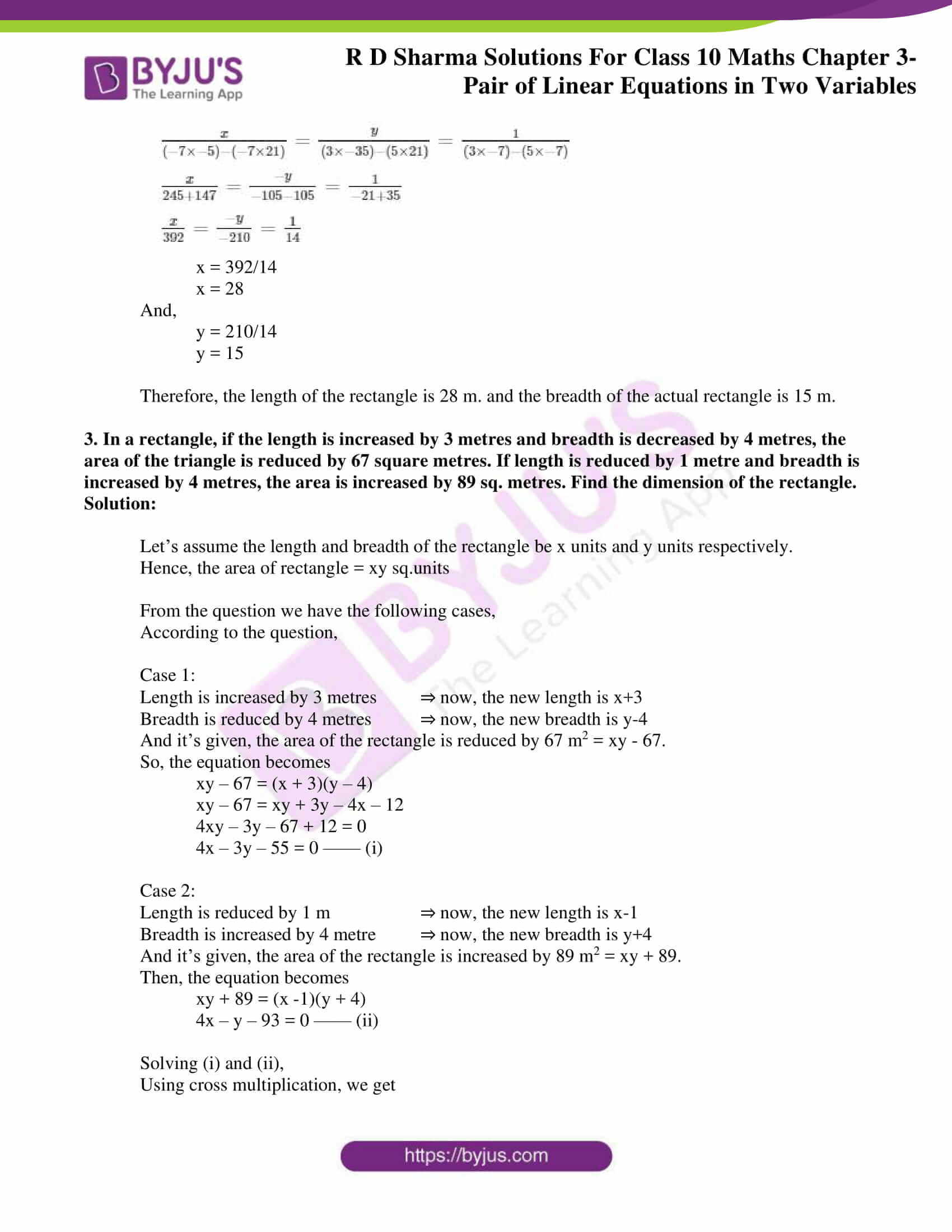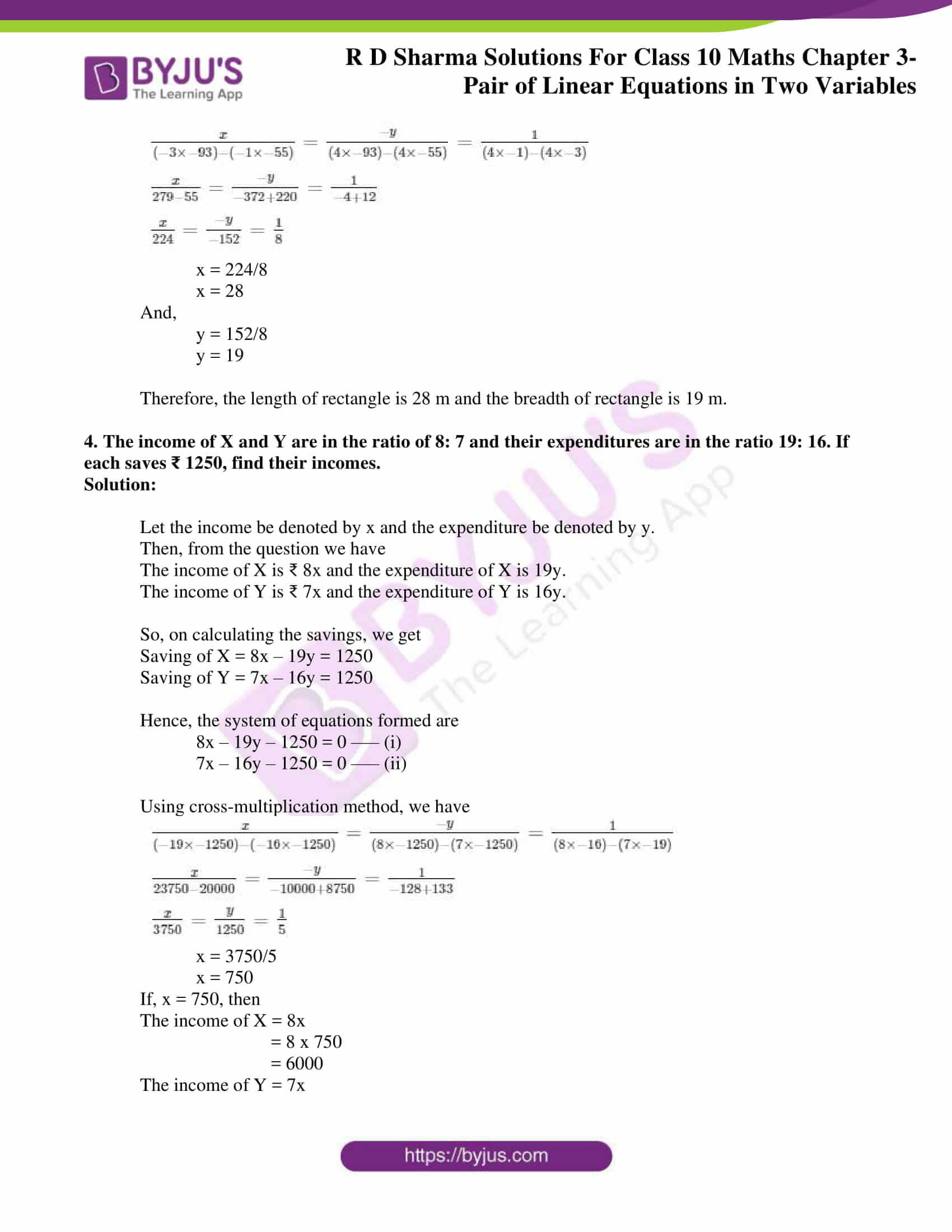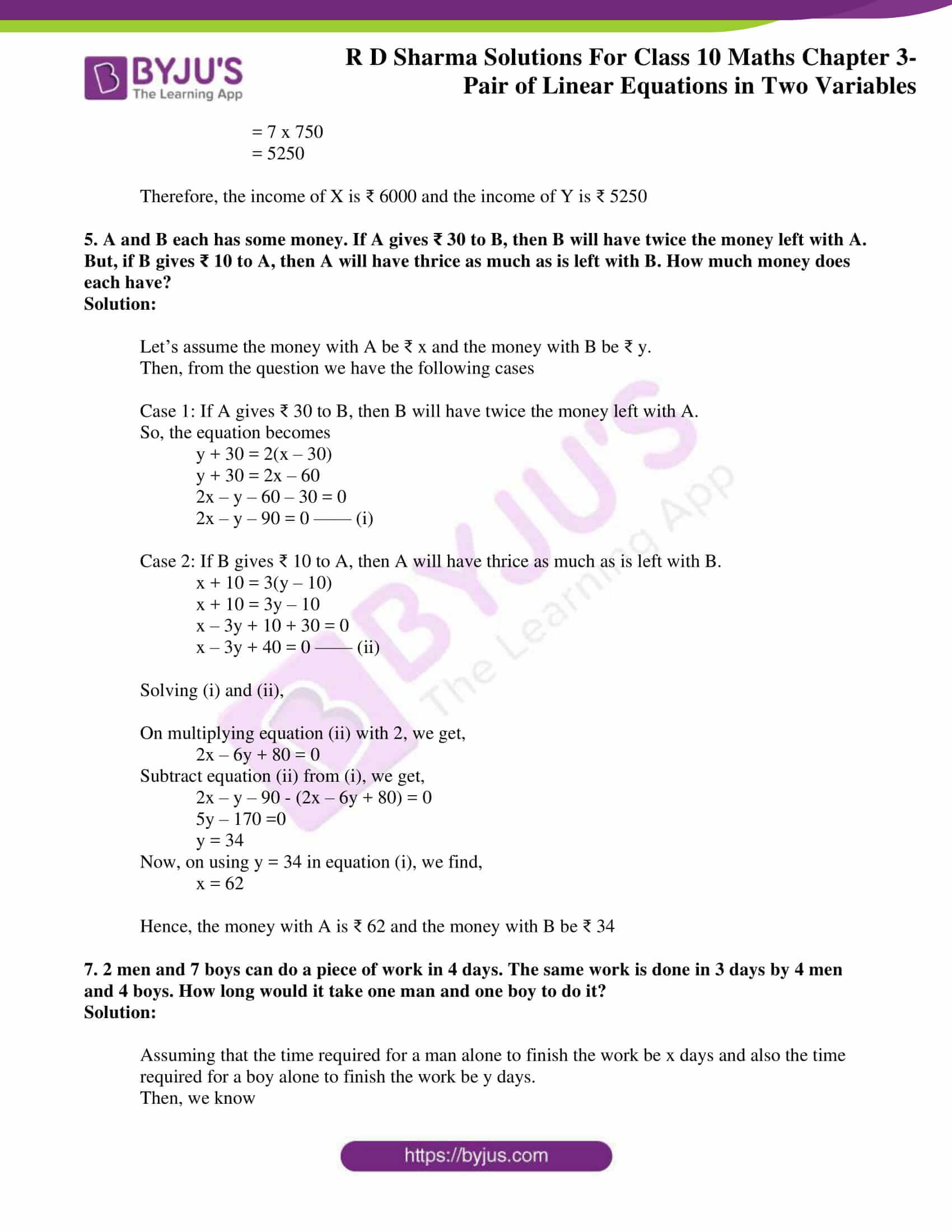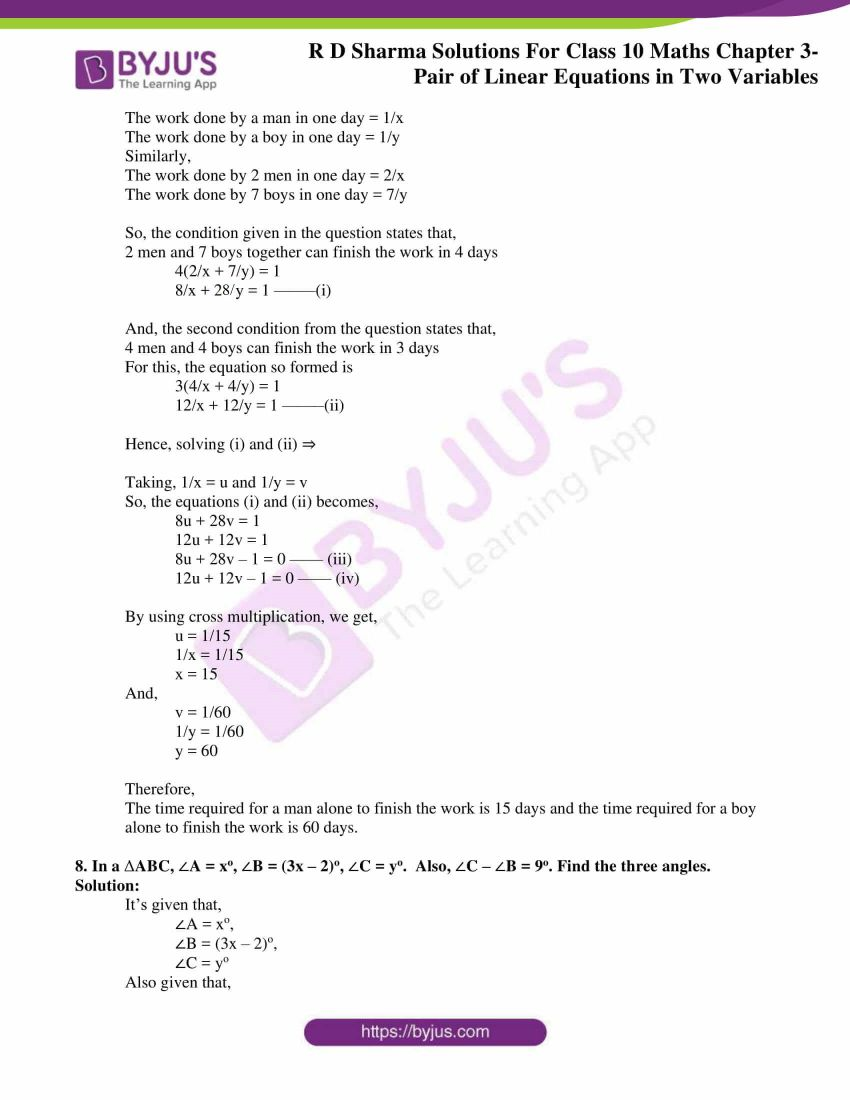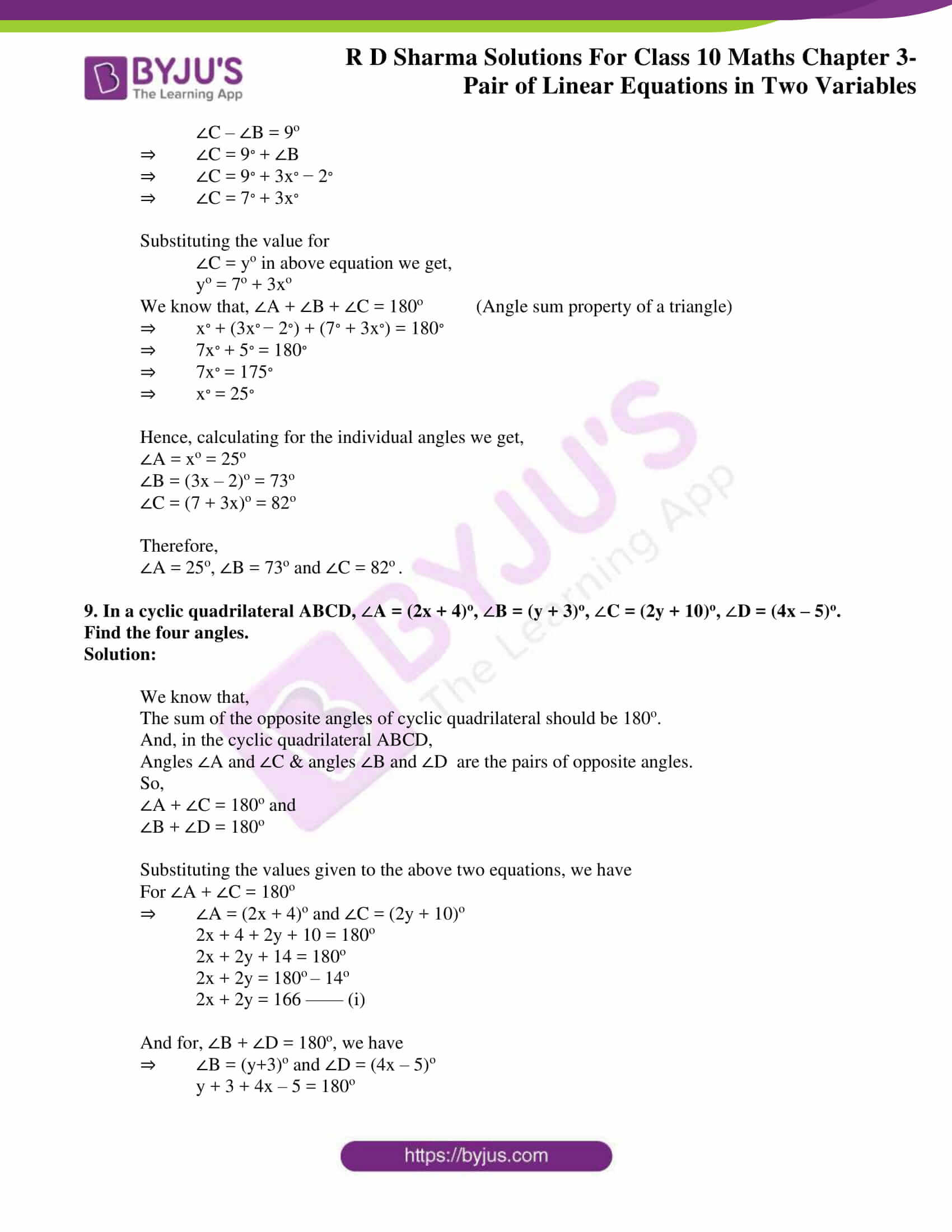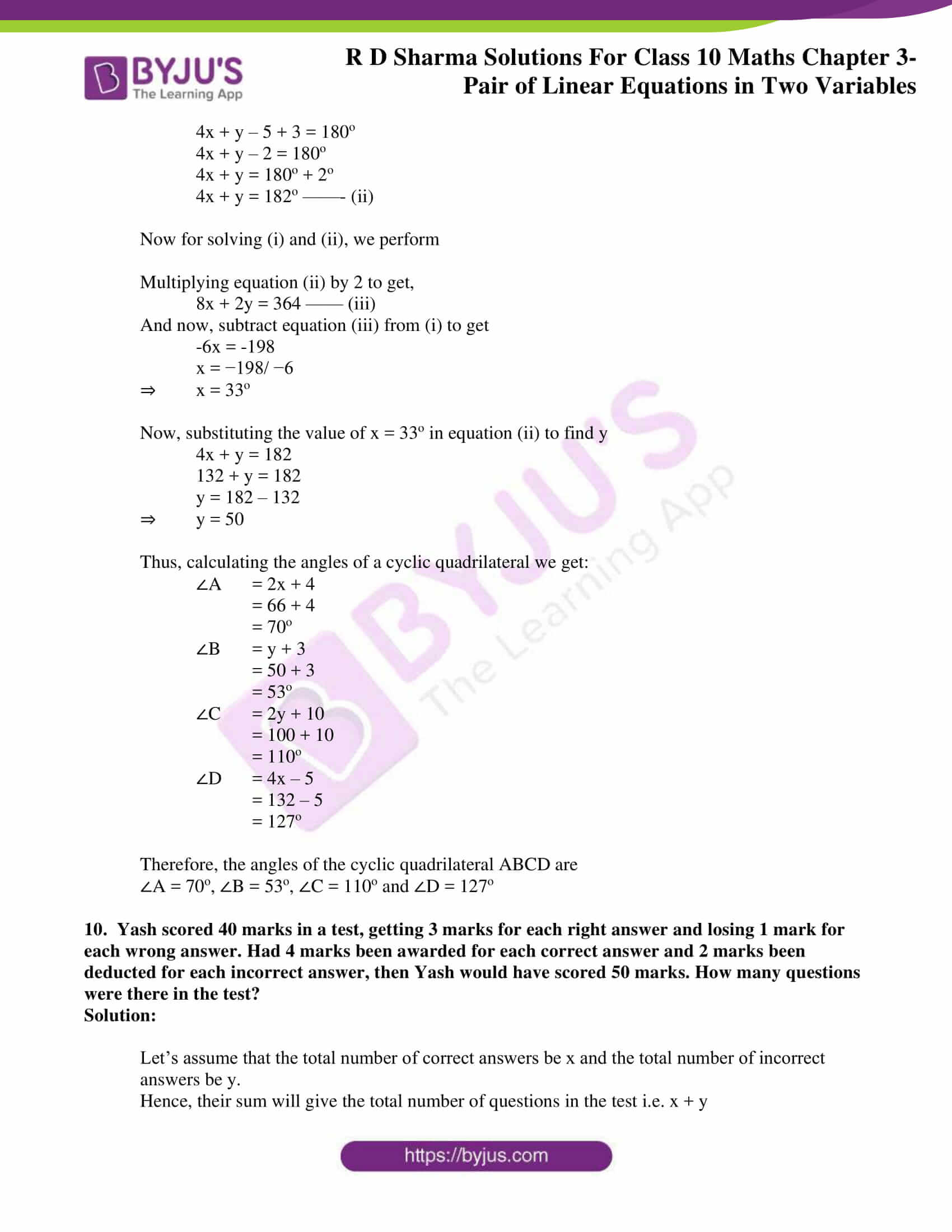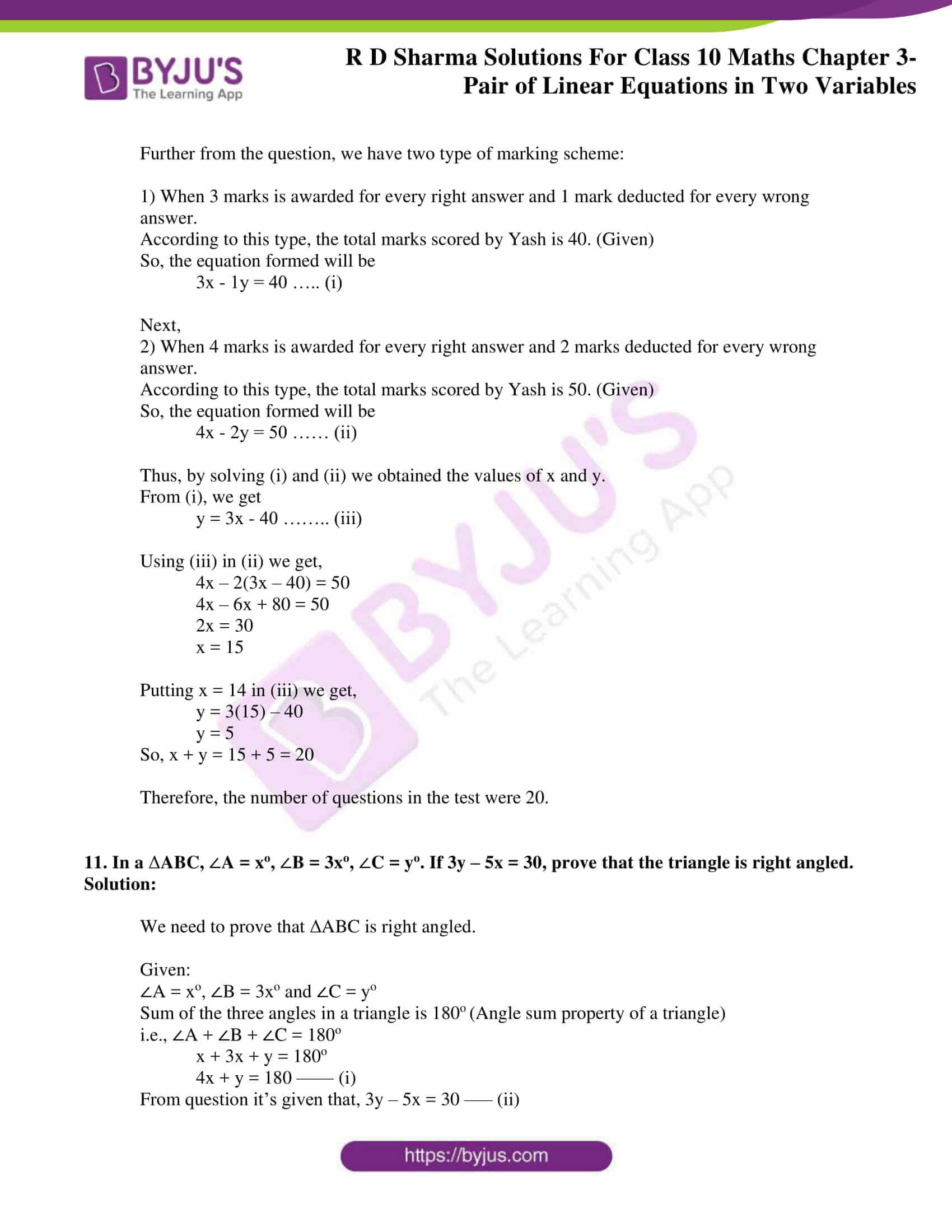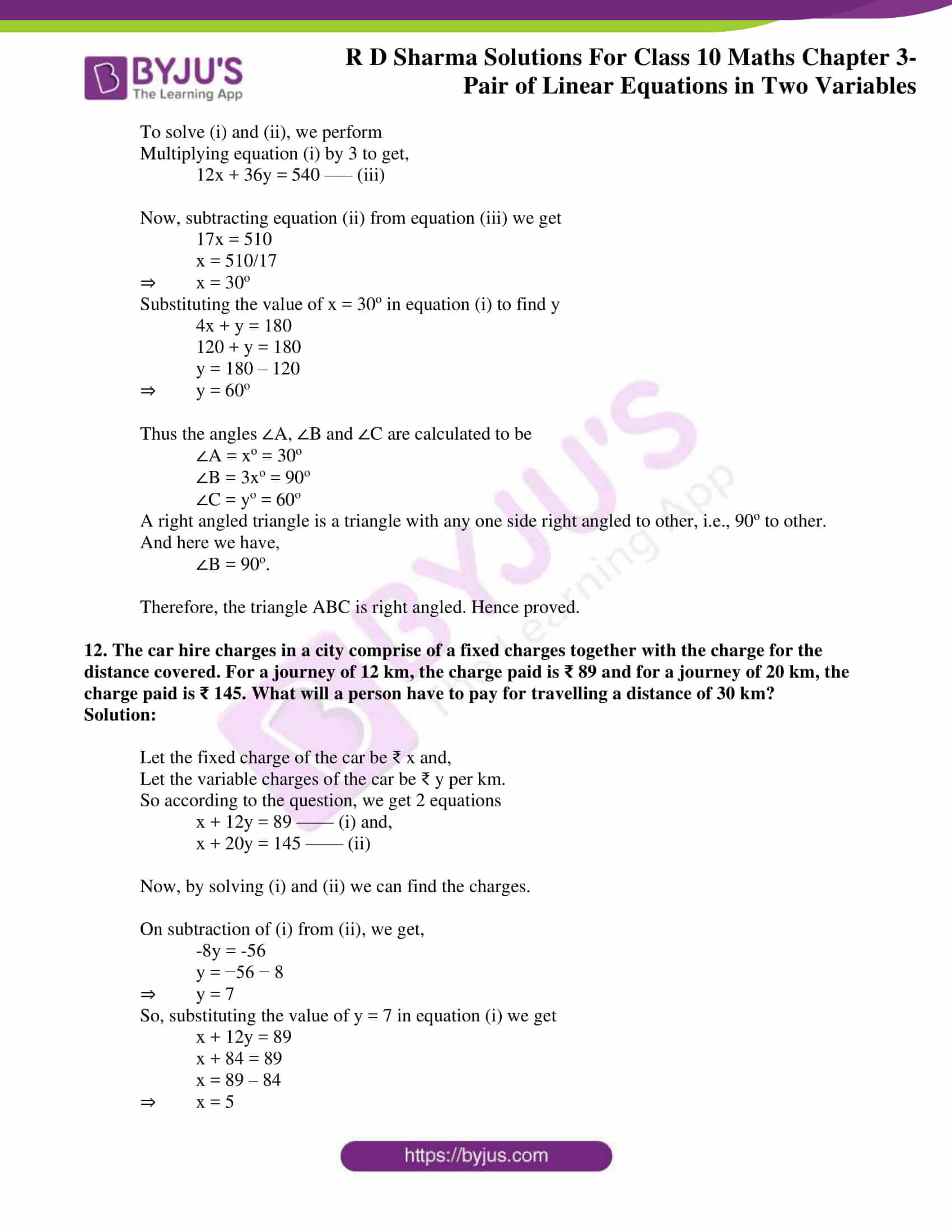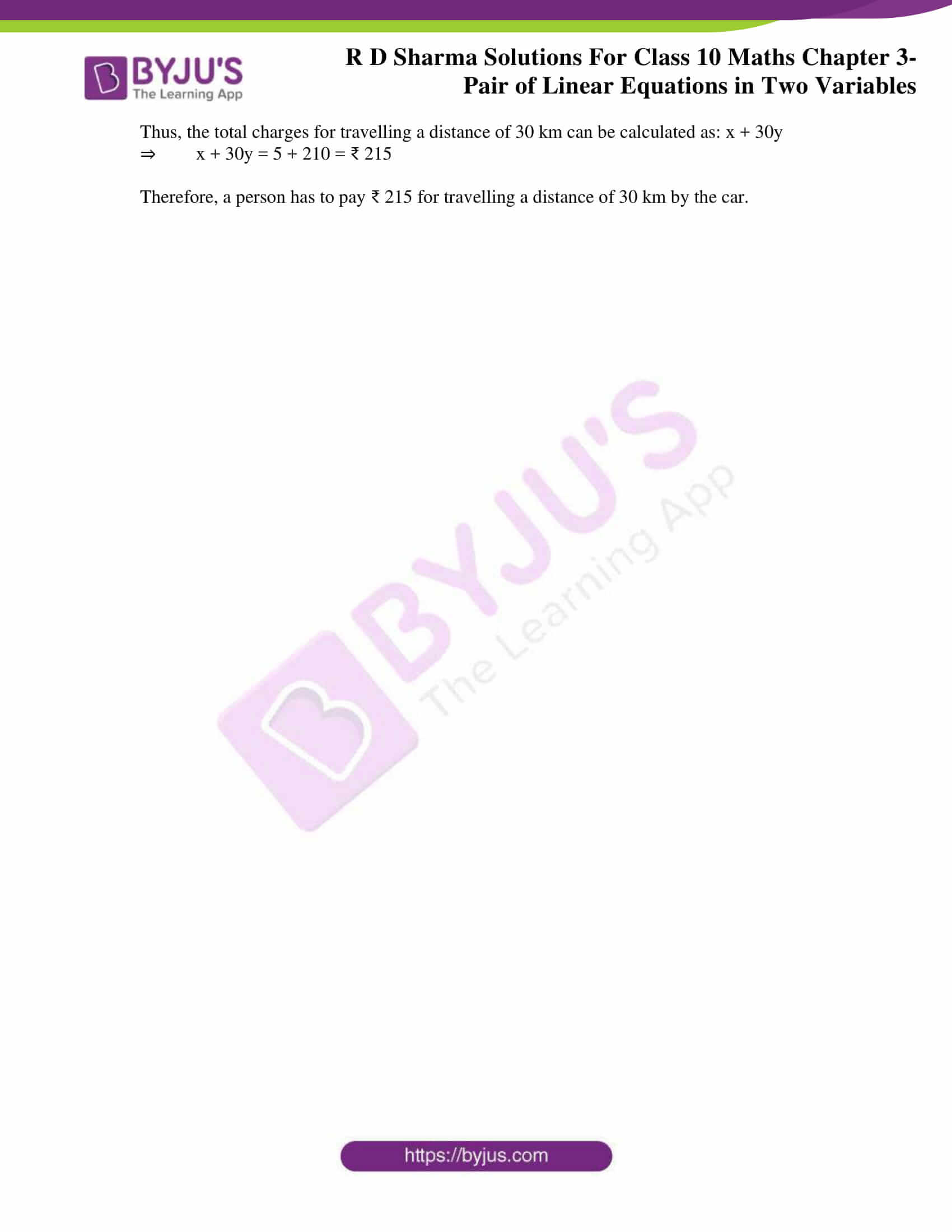### Access RD Sharma Solutions for Class 10 Maths Chapter 3 Pair of Linear Equations In Two Variables Exercise 3.11

1. If in a rectangle, the length is increased and breadth reduced each by 2 units, the area is reduced by 28 square units. If, however the length is reduced by 1 unit and the breadth increased by 2 units, the area increases by 33 square units. Find the area of the rectangle.

Solution:

Let’s assume the length and breadth of the rectangle be x units and y units, respectively.

Hence, the area of rectangle = xy sq. units

From the question we have the following cases,

Case 1:

Length is increased by 2 units ⇒ now, the new length is x+2 units

Breadth is reduced by 2 units ⇒ now, the new breadth is y-2 units

And it’s given that the area is reduced by 28 square units i.e., = xy – 28

So, the equation becomes

(x+2)(y−2) = xy − 28

⇒ xy − 2x + 2y – 4 = xy − 28

⇒ −2x + 2y – 4 + 28 = 0

⇒ −2x + 2y + 24 = 0

⇒ 2x − 2y – 24 = 0 ……… (i)

Case 2:

Length is reduced by 1 unit ⇒ now, the new length is x-1 units

Breadth is increased by 2 units ⇒ now, the new breadth is y+2 units

And, it’s given that the area is increased by 33 square units i.e. = i.e. = xy + 33

So, the equation becomes

(x−1)(y+2) = xy + 33

⇒ xy + 2x – y – 2 = x + 33

⇒ 2x – y − 2 − 33 = 0

⇒ 2x – y −35 = 0 ……….. (ii)

Solving (i) and (ii),

By using cross multiplication, we get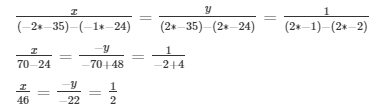x = 46/2

x = 23

And,

y = 22/2

y = 11

Hence,

The length of the rectangle is 23 units.

The breadth of the rectangle is 11 units.

So, the area of the actual rectangle = length x breadth,

= x×y

= 23 x 11

= 253 sq. units

Therefore, the area of rectangle is 253 sq. units.

2. The area of a rectangle remains the same if the length is increased by 7 metres and the breadth is decreased by 3 metres. The area remains unaffected if the length is decreased by 7 metres and the breadth is increased by 5 metres. Find the dimensions of the rectangle.

Solution:

Let’s assume the length and breadth of the rectangle be x units and y units, respectively.

Hence, the area of rectangle = xy sq.units

From the question we have the following cases,

Case 1

Length is increased by 7 metres ⇒ now, the new length is x+7

Breadth is decreased by 3 metres ⇒ now, the new breadth is y-3

And it’s given, the area of the rectangle remains the same i.e. = xy.

So, the equation becomes

xy = (x+7)(y−3)

xy = xy + 7y − 3x − 21

3x – 7y + 21 = 0 ………. (i)

Case 2:

Length is decreased by 7 metres ⇒ now, the new length is x-7

Breadth is increased by 5 metres ⇒ now, the new breadth is y+5

And it’s given that, the area of the rectangle still remains the same i.e. = xy.

So, the equation becomes

xy = (x−7)(y+5)

xy = xy − 7y + 5x − 35

5x – 7y – 35 = 0 ………. (ii)

Solving (i) and (ii),

By using cross-multiplication, we get,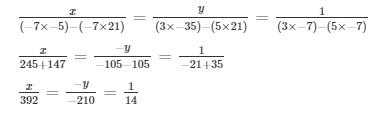x = 392/14

x = 28

And,

y = 210/14

y = 15

Therefore, the length of the rectangle is 28 m. and the breadth of the actual rectangle is 15 m.

3. In a rectangle, if the length is increased by 3 metres and breadth is decreased by 4 metres, the area of the triangle is reduced by 67 square metres. If length is reduced by 1 metre and breadth is increased by 4 metres, the area is increased by 89 sq. metres. Find the dimension of the rectangle.

Solution:

Let’s assume the length and breadth of the rectangle be x units and y units, respectively.

Hence, the area of rectangle = xy sq.units

From the question we have the following cases,

According to the question,

Case 1:

Length is increased by 3 metres ⇒ now, the new length is x+3

Breadth is reduced by 4 metres ⇒ now, the new breadth is y-4

And it’s given, the area of the rectangle is reduced by 67 m2 = xy – 67.

So, the equation becomes

xy – 67 = (x + 3)(y – 4)

xy – 67 = xy + 3y – 4x – 12

4xy – 3y – 67 + 12 = 0

4x – 3y – 55 = 0 —— (i)

Case 2:

Length is reduced by 1 m ⇒ now, the new length is x-1

Breadth is increased by 4 metre ⇒ now, the new breadth is y+4

And it’s given, the area of the rectangle is increased by 89 m2 = xy + 89.

Then, the equation becomes

xy + 89 = (x -1)(y + 4)

4x – y – 93 = 0 —— (ii)

Solving (i) and (ii),

Using cross multiplication, we get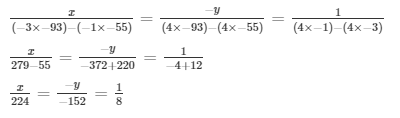x = 224/8

x = 28

And,

y = 152/8

y = 19

Therefore, the length of rectangle is 28 m and the breadth of rectangle is 19 m.

4. The income of X and Y are in the ratio of 8: 7 and their expenditures are in the ratio 19: 16. If each saves ₹ 1250, find their incomes.

Solution:

Let the income be denoted by x and the expenditure be denoted by y.

Then, from the question we have

The income of X is ₹ 8x and the expenditure of X is 19y.

The income of Y is ₹ 7x and the expenditure of Y is 16y.

So, on calculating the savings, we get

Saving of X = 8x – 19y = 1250

Saving of Y = 7x – 16y = 1250

Hence, the system of equations formed are

8x – 19y – 1250 = 0 —– (i)

7x – 16y – 1250 = 0 —– (ii)

Using cross-multiplication method, we have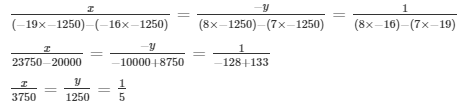x = 3750/5

x = 750

If, x = 750, then

The income of X = 8x

= 8 x 750

= 6000

The income of Y = 7x

= 7 x 750

= 5250

Therefore, the income of X is ₹ 6000 and the income of Y is ₹ 5250

5. A and B each has some money. If A gives ₹ 30 to B, then B will have twice the money left with A. But, if B gives ₹ 10 to A, then A will have thrice as much as is left with B. How much money does each have?

Solution:

Let’s assume the money with A be ₹ x and the money with B be ₹ y.

Then, from the question we have the following cases

Case 1: If A gives ₹ 30 to B, then B will have twice the money left with A.

So, the equation becomes

y + 30 = 2(x – 30)

y + 30 = 2x – 60

2x – y – 60 – 30 = 0

2x – y – 90 = 0 —— (i)

Case 2: If B gives ₹ 10 to A, then A will have thrice as much as is left with B.

x + 10 = 3(y – 10)

x + 10 = 3y – 10

x – 3y + 10 + 30 = 0

x – 3y + 40 = 0 —— (ii)

Solving (i) and (ii),

On multiplying equation (ii) with 2, we get,

2x – 6y + 80 = 0

Subtract equation (ii) from (i), we get,

2x – y – 90 – (2x – 6y + 80) = 0

5y – 170 =0

y = 34

Now, on using y = 34 in equation (i), we find,

x = 62

Hence, the money with A is ₹ 62 and the money with B be ₹ 34

7. 2 men and 7 boys can do a piece of work in 4 days. The same work is done in 3 days by 4 men and 4 boys. How long would it take one man and one boy to do it?

Solution:

Assuming that the time required for a man alone to finish the work be x days and also the time required for a boy alone to finish the work be y days.

Then, we know

The work done by a man in one day = 1/x

The work done by a boy in one day = 1/y

Similarly,

The work done by 2 men in one day = 2/x

The work done by 7 boys in one day = 7/y

So, the condition given in the question states that,

2 men and 7 boys together can finish the work in 4 days

4(2/x + 7/y) = 1

8/x + 28/y = 1 ——–(i)

And, the second condition from the question states that,

4 men and 4 boys can finish the work in 3 days

For this, the equation so formed is

3(4/x + 4/y) = 1

12/x + 12/y = 1 ——–(ii)

Hence, solving (i) and (ii) ⇒

Taking, 1/x = u and 1/y = v

So, the equations (i) and (ii) becomes,

8u + 28v = 1

12u + 12v = 1

8u + 28v – 1 = 0 —— (iii)

12u + 12v – 1 = 0 —— (iv)

By using cross multiplication, we get,

u = 1/15

1/x = 1/15

x = 15

And,

v = 1/60

1/y = 1/60

y = 60

Therefore,

The time required for a man alone to finish the work is 15 days and the time required for a boy alone to finish the work is 60 days.

8. In a ΔABC, A = xo, B = (3x – 2)o, C = yo. Also, C –B = 9o. Find the three angles.

Solution:

It’s given that,

∠A = xo,

∠B = (3x – 2)o,

∠C = yo

Also given that,

∠C – ∠B = 9o

⇒ ∠C = 9 + ∠B

⇒ ∠C = 9 + 3x − 2

⇒ ∠C = 7 + 3x

Substituting the value for

∠C = yo in above equation we get,

yo = 7o + 3xo

We know that, ∠A + ∠B + ∠C = 180o (Angle sum property of a triangle)

⇒ x + (3x− 2) + (7 + 3x) = 180

⇒ 7x + 5 = 180

⇒ 7x = 175

⇒ x = 25

Hence, calculating for the individual angles we get,

∠A = xo = 25o

∠B = (3x – 2)o = 73o

∠C = (7 + 3x)o = 82o

Therefore,

∠A = 25o, ∠B = 73o and ∠C = 82o .

9. In a cyclic quadrilateral ABCD, A = (2x + 4)o, B = (y + 3)o, C = (2y + 10)o, D = (4x – 5)o. Find the four angles.

Solution:

We know that,

The sum of the opposite angles of cyclic quadrilateral should be 180o.

And, in the cyclic quadrilateral ABCD,

Angles ∠A and ∠C & angles ∠B and ∠D are the pairs of opposite angles.

So,

∠A + ∠C = 180o and

∠B + ∠D = 180o

Substituting the values given to the above two equations, we have

For ∠A + ∠C = 180o

⇒ ∠A = (2x + 4)o and ∠C = (2y + 10)o

2x + 4 + 2y + 10 = 180o

2x + 2y + 14 = 180o

2x + 2y = 180– 14o

2x + 2y = 166 —— (i)

And for, ∠B + ∠D = 180o, we have

⇒ ∠B = (y+3)o and ∠D = (4x – 5)o

y + 3 + 4x – 5 = 180o

4x + y – 5 + 3 = 180o

4x + y – 2 = 180o

4x + y = 180o + 2o

4x + y = 182o ——- (ii)

Now for solving (i) and (ii), we perform

Multiplying equation (ii) by 2 to get,

8x + 2y = 364 —— (iii)

And now, subtract equation (iii) from (i) to get

-6x = -198

x = −198/ −6

⇒ x = 33o

Now, substituting the value of x = 33o in equation (ii) to find y

4x + y = 182

132 + y = 182

y = 182 – 132

⇒ y = 50

Thus, calculating the angles of a cyclic quadrilateral we get:

∠A = 2x + 4

= 66 + 4

= 70o

∠B = y + 3

= 50 + 3

= 53o

∠C = 2y + 10

= 100 + 10

= 110o

∠D = 4x – 5

= 132 – 5

= 127o

Therefore, the angles of the cyclic quadrilateral ABCD are

∠A = 70o, ∠B = 53o, ∠C = 110o and ∠D = 127o

10. Yash scored 40 marks in a test, getting 3 marks for each right answer and losing 1 mark for each wrong answer. Had 4 marks been awarded for each correct answer and 2 marks been deducted for each incorrect answer, then Yash would have scored 50 marks. How many questions were there in the test?

Solution:

Let’s assume that the total number of correct answers be x and the total number of incorrect answers be y.

Hence, their sum will give the total number of questions in the test i.e. x + y

Further from the question, we have two type of marking scheme:

1) When 3 marks is awarded for every right answer and 1 mark deducted for every wrong answer.

According to this type, the total marks scored by Yash is 40. (Given)

So, the equation formed will be

3x – 1y = 40 ….. (i)

Next,

2) When 4 marks is awarded for every right answer and 2 marks deducted for every wrong answer.

According to this type, the total marks scored by Yash is 50. (Given)

So, the equation formed will be

4x – 2y = 50 …… (ii)

Thus, by solving (i) and (ii) we obtained the values of x and y.

From (i), we get

y = 3x – 40 …….. (iii)

Using (iii) in (ii) we get,

4x – 2(3x – 40) = 50

4x – 6x + 80 = 50

2x = 30

x = 15

Putting x = 14 in (iii) we get,

y = 3(15) – 40

y = 5

So, x + y = 15 + 5 = 20

Therefore, the number of questions in the test were 20.

11. In a ΔABC, A = xo, B = 3xo, C = yo. If 3y – 5x = 30, prove that the triangle is right-angled.

Solution:

We need to prove that ΔABC is right-angled.

Given:

∠A = xo, ∠B = 3xo and ∠C = yo

Sum of the three angles in a triangle is 180o (Angle sum property of a triangle)

i.e., ∠A + ∠B + ∠C = 180o

x + 3x + y = 180o

4x + y = 180 —— (i)

From question it’s given that, 3y – 5x = 30 —– (ii)

To solve (i) and (ii), we perform

Multiplying equation (i) by 3 to get,

12x + 36y = 540 —– (iii)

Now, subtracting equation (ii) from equation (iii) we get

17x = 510

x = 510/17

⇒ x = 30o

Substituting the value of x = 30o in equation (i) to find y

4x + y = 180

120 + y = 180

y = 180 – 120

⇒ y = 60o

Thus the angles ∠A, ∠B and ∠C are calculated to be

∠A = xo = 30o

∠B = 3xo = 90o

∠C = yo = 60o

A right angled triangle is a triangle with any one side right angled to other, i.e., 90o to other.

And here we have,

∠B = 90o.

Therefore, the triangle ABC is right angled. Hence proved.

12. The car hire charges in a city comprise of a fixed charges together with the charge for the distance covered. For a journey of 12 km, the charge paid is ₹ 89 and for a journey of 20 km, the charge paid is ₹ 145. What will a person have to pay for travelling a distance of 30 km?

Solution:

Let the fixed charge of the car be ₹ x and,

Let the variable charges of the car be ₹ y per km.

So according to the question, we get 2 equations

x + 12y = 89 —— (i) and,

x + 20y = 145 —— (ii)

Now, by solving (i) and (ii) we can find the charges.

On subtraction of (i) from (ii), we get,

-8y = -56

y = −56 − 8

⇒ y = 7

So, substituting the value of y = 7 in equation (i) we get

x + 12y = 89

x + 84 = 89

x = 89 – 84

⇒ x = 5

Thus, the total charges for travelling a distance of 30 km can be calculated as: x + 30y

⇒ x + 30y = 5 + 210 = ₹ 215

Therefore, a person has to pay ₹ 215 for travelling a distance of 30 km by the car.